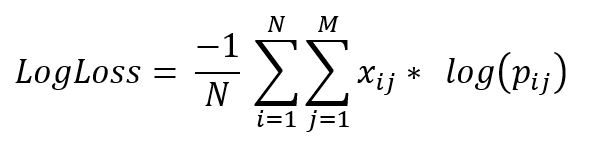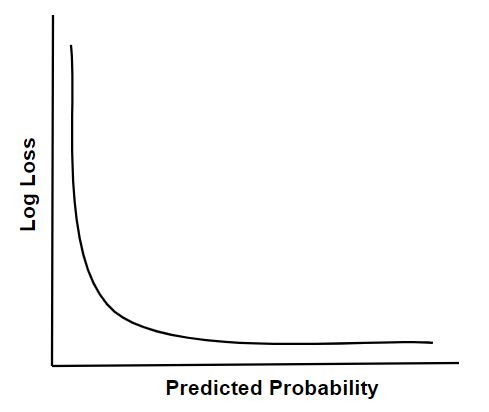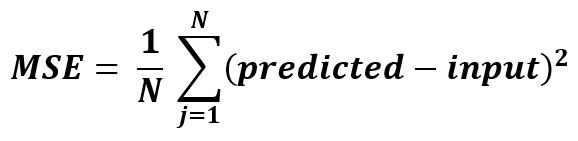# Log Loss

It is the evaluation measure to check the performance of the classification model. It measures the amount of divergence of predicted probability with the actual label. So lesser the log loss value, more the perfectness of model. For a perfect model, log loss value = 0. For instance, as accuracy is the count of correct predictions i.e. the prediction that matches the actual label, Log Loss value is the measure of uncertainty of our predicted labels based on how it varies from the actual label.```where,
N  : no. of samples.
M  : no. of attributes.
yij : indicates whether ith sample belongs to jth class or not.
pij : indicates probability of ith sample belonging to jth class.
```Implementation of LogLoss using sklearn

 `from` `sklearn.metrics ``import` `log_loss: ` ` `  `LogLoss ``=` `log_loss(y_true, y_pred, eps ``=` `1e``-``15``, ` `    ``normalize ``=` `True``, sample_weight ``=` `None``, labels ``=` `None``) `

# Mean Squared Error

It is simply the average of the square of the difference between the original values and the predicted values.Implementation of Mean Squared Error using sklearn

 `from` `sklearn.metrics ``import` `mean_squared_error ` ` `  `MSE ``=` `mean_squared_error(y_true, y_pred) `

My Personal Notes arrow_drop_upAspire to Inspire before I expire

If you like GeeksforGeeks and would like to contribute, you can also write an article using contribute.geeksforgeeks.org or mail your article to contribute@geeksforgeeks.org. See your article appearing on the GeeksforGeeks main page and help other Geeks.

Please Improve this article if you find anything incorrect by clicking on the "Improve Article" button below.